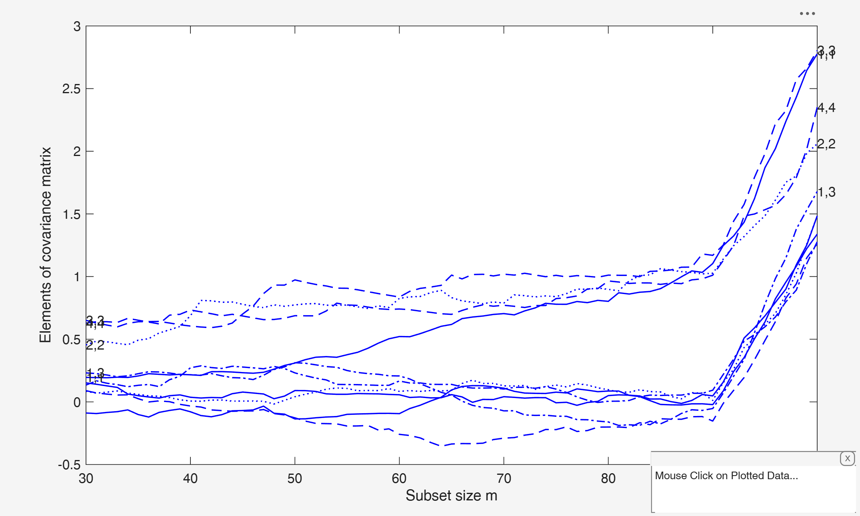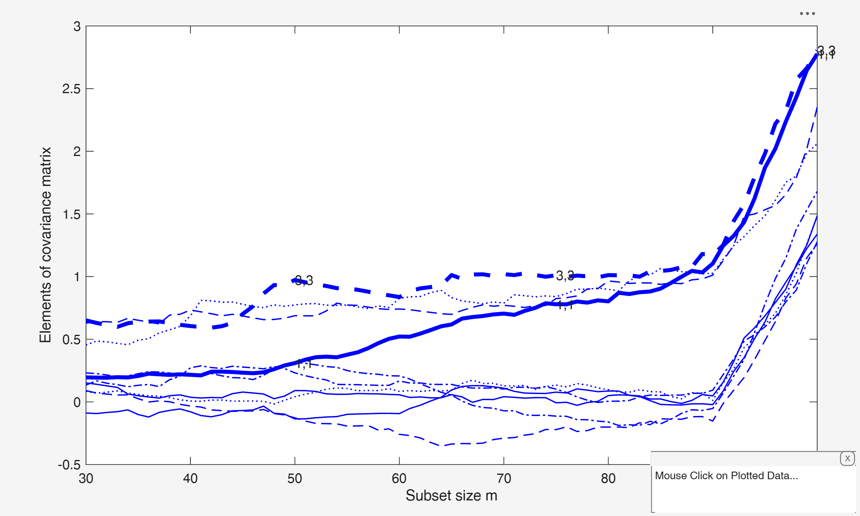covplot

covplot plots the trajectories of the elements of the covariance (correlation) matrix monitored

Description

covplot(out) covplot with all default options.

covplot(out, Name, Value) covplot with optional arguments.

Examples

expand allcovplot with all default options.

generate input structure for malfwdplot

n=100;
p=4;
state1=141243498;
randn('state', state1);
Y=randn(n,p);
kk=[1:10];
Y(kk,:)=Y(kk,:)+4;
[fre]=unibiv(Y);
m0=20;
bs=fre(1:m0,1);
[out]=FSMeda(Y,bs,'init',30);
% Produce monitoring covariances plot with all the default options
covplot(out)
Warning: Support for GPU devices with Compute Capability 5.2 will be removed in
a future MATLAB release. For more information on GPU support, see <a
href="matlab:web('http://www.mathworks.com/help/parallel-computing/gpu-support-by-release.html','-browser')">GPU
Support by Release</a>.covplot with optional arguments.

Example of the use of some options inside structure standard.

n=100;
p=4;
state1=141243498;
randn('state', state1);
Y=randn(n,p);
kk=[1:10];
Y(kk,:)=Y(kk,:)+4;
[fre]=unibiv(Y);
m0=20;
bs=fre(1:m0,1);
[out]=FSMeda(Y,bs,'init',30);
% Initialize structure standard
standard=struct;
standard.LineStyle={'-';'-.';'--'};
% Specify the line width
standard.LineWidth=0.5;
covplot(out,'standard',standard)

Related Examples

expand all

Example of the use of some options inside structure fground.

n=100;
p=4;
state1=141243498;
randn('state', state1);
Y=randn(n,p);
kk=[1:10];
Y(kk,:)=Y(kk,:)+4;
[fre]=unibiv(Y);
m0=20;
bs=fre(1:m0,1);
[out]=FSMeda(Y,bs,'init',30);
% Initialize structure fground
fground = struct;
% Specify the number of trajectories which have to be highlighted
fground.funit=2;
% Specify the steps in which labels have to be put
n=size(Y,1);
fground.flabstep=[n/2 n*0.75 n+0.5];
% Specify the line width of the highlighted trajectories
fground.LineWidth=3;
% Produce a monitoring residuals plot in which labels are put for units
% [2 5 20 23 35 45] in steps [n/2 n*0.75 n+0.5] of the search
covplot(out,'fground',fground)Example of the use of option tag.

n=100;
p=4;
state1=141243498;
randn('state', state1);
Y=randn(n,p);
kk=[1:10];
Y(kk,:)=Y(kk,:)+4;
[fre]=unibiv(Y);
m0=20;
bs=fre(1:m0,1);
[out]=FSMeda(Y,bs,'init',30);
% Initialize structure fground
fground = struct;
% Specify the number of trajectories which have to be highlighted
fground.funit=2;
% Specify the steps in which labels have to be put
n=size(Y,1);
fground.flabstep=[n/2 n*0.75 n+0.5];
% Specify the line width of the highlighted trajectories
fground.LineWidth=3;
covplot(out,'fground',fground,'tag','pl_mycov')Input Arguments

out — Data to plot. Structure.

Structure containing the following fields

Value Description
S2cov

(n-init+1) x (v*(v+1)/2+1) matrix containing the monitoring of the elements of the covariance matrix in each step of the forward search:

1st col = fwd search index (from init to n);

2nd col = monitoring of S(1,1);

3rd col = monitoring of S(1,2);

...;

(compulsory argument).

Un

matrix containing the order of entry of each unit (necessary if datatooltip is true).

Y

n x v data matrix; n observations and v variables.

Data Types: struct

Name-Value Pair Arguments

Specify optional comma-separated pairs of Name,Value arguments. Name is the argument name and Value is the corresponding value. Name must appear inside single quotes (' '). You can specify several name and value pair arguments in any order as Name1,Value1,...,NameN,ValueN.

Example: 'standard',standard , 'fground',fground , 'tag','pl_mycov' , 'datatooltip',''

standard —Appearance of the plot.structure.

Structure which defines the appearance of the plot in terms of xlim, ylim, axes labels and their font size style, color of the lines, etc.

The structure contains the following fields:

Value Description
SizeAxesNum

scalar specifying the fontsize of the axes numbers. Default value is 10.

xlim

two elements vector with minimum and maximum of the x axis. Default value is '' (automatic scale).

ylim

two elements vector with minimum and maximum of the y axis. Default value is '' (automatic scale).

titl

a label for the title (default: '').

labx

a label for the x-axis (default: 'Subset size m').

laby

a label for the y-axis (default: 'Elements of the correlation (covariance) matrix)

SizeAxesLab

Scalar specifying the fontsize of the labels of the axes. Default value is 12.

LineWidth

scalar specifying line width for the trajectories.

Color

cell array of strings containing the colors to be used for the standard units.

If length(Color)=1 the same color will be used for all units.

If length(Color)=2 half of the trajectories will appear with Color{1} and the other half with Color{2}. And so on with 3 cell elements, etc.

LineStyle

cell containing the line types.

Example: 'standard',standard

Data Types: structure

fground —Trajectories in foregroud.structure.

Structure which defines the trajectories in foregroud, that is which trajectories are highlighted and how they are plotted to be distinguishable from the others.

It is possible to control the label, the width, the color, the line type and the marker of the highlighted covariances. The structure fground contains the following fields:

Value Description
fthresh

(alternative to funit) numeric vector of length 1 or 2 which specifies the highlighted trajectories.

If length(fthresh)=1 the highlighted trajectories are those of units that throughtout the search had at leat once a covariance greater (in absolute value) than thresh. The default value of fthresh is Inf.

If length(fthresh)=2 the highlighted trajectories are those of units that throughtout the search had a covariance at leat once bigger than fthresh(2) or smaller than fthresh(1).

funit

(alternative to fthresh) scalar containing the number of trajectories of the covariances to be highlighted. For example if funit=5 the trajectories with the 5 highest values of the covariances are highlighted.

Notice that if funit is supplied, fthresh is ignored. The default value of funit is 5.

flabstep

numeric vector which specifies the steps of the search where to put labels for the highlighted trajectories (units). The default is to put the labels at the initial and final steps of the search, that is fground.flabstep=[m0 n].

flabstep='' means no label.

LineWidth

scalar specifying line width for the highlighted trajectories (units). Default is 1.

Color

cell array of strings containing the colors to be used for the highlighted trajectories (units).

If length(scolor)==1 the same color will be used for all highlighted units. If for example length(scolor)=2 and there are 6 highlighted units, 3 highlighted trajectories appear with selunitcolor{1} and 3 highlighted trajectories with selunitcolor{2}.

LineStyle

cell containing the line type of the highlighted trajectories.

fmark

scalar controlling whether to plot highlighted trajectories as markers. If 1 each trajectory is plotted using a different marker else (default) no marker is used.

Example: 'fground',fground

Data Types: structure

tag —Handle of the plot.string.

String which identifies the handle of the plot which is about to be created. The default is to use tag 'pl_cov'. Note that if the program finds a plot which has a tag equal to the one specified by the user, then the output of the new plot overwrites the existing one in the same window else a new window is created.

Example: 'tag','pl_mycov'

Data Types: string

datatooltip —Information about the unit selected.empty value | structure.

The default is datatooltip=1.

If datatooltip is not empty the user can use the mouse in order to have information about the unit selected, the step in which the unit enters the search and the associated label.

If datatooltip is a structure, it is possible to control the aspect of the data cursor (see function datacursormode for more details or the examples below).

The default options of the structure are DisplayStyle='Window' and SnapToDataVertex='on'.

Example: 'datatooltip',''

Data Types: Empty value or structure.

Output Arguments

Atkinson, A.C., Riani, M. and Cerioli, A. (2004), "Exploring multivariate data with the forward search", Springer Verlag, New York.